# Area of Quadrilateral

• Difficulty Level : Expert
• Last Updated : 21 Sep, 2022

Area of a quadrilateral is the space inside the boundary of a quadrilateral. A quadrilateral can be defined as a closed two-dimensional shape that has four sides or edges, and also four corners or vertices. In mensuration, the shape of objects is classified based on the number of sides of the quadrilateral. Quadrilaterals are categorized into two categories, regular quadrilaterals, and irregular quadrilaterals. A regular quadrilateral is a quadrilateral that has all sides of equal length. An irregular quadrilateral is a quadrilateral with all the sides not equal.

## What is a Quadrilateral?

A quadrilateral is a polygon with four sides. A closed two-dimensional figure, formed by joining the four points among which three points are non-collinear points is called a quadrilateral. A quadrilateral has four sides, four angles, and four vertices. The sides of the quadrilateral may or may not be equal.

As defined above any polygon having four sides is termed a quadrilateral. Various types of quadrilateral are

• Rectangle
• Square
• Rhombus
• Parallelogram
• Trapezium
• Kite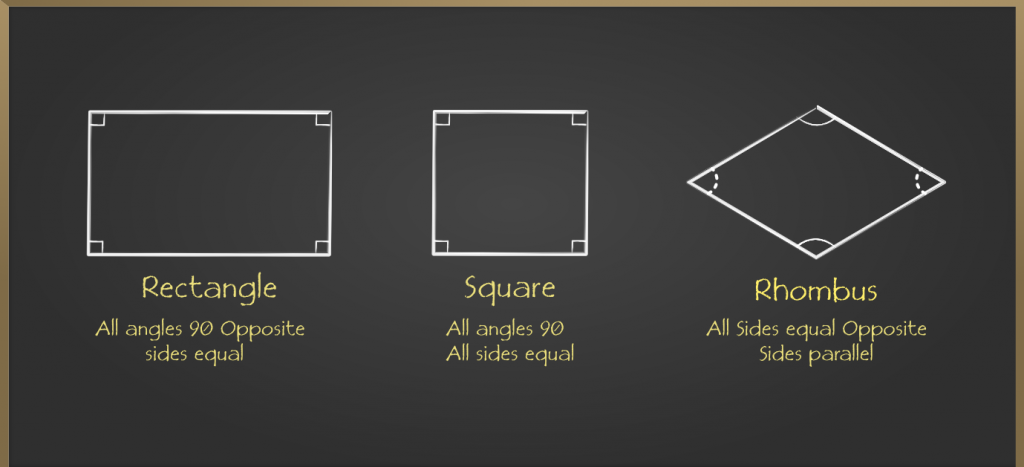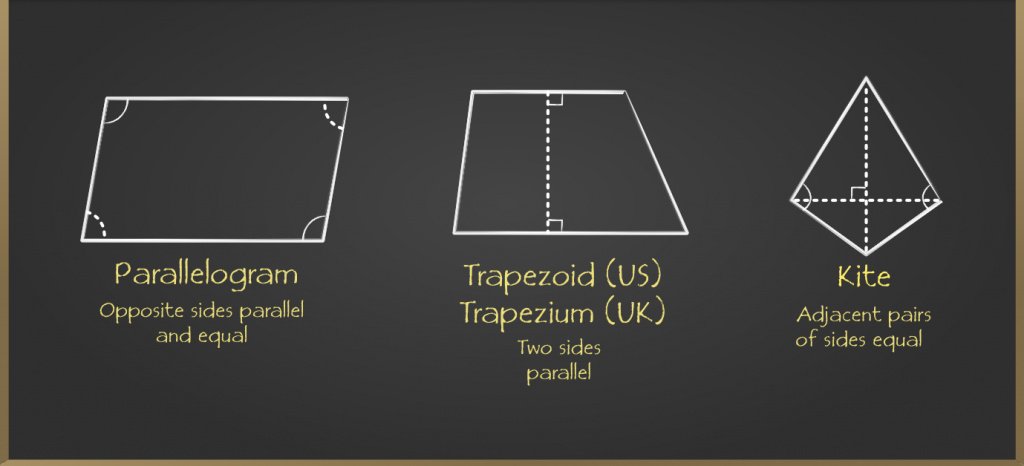## Properties of General Quadrilateral

• A closed figure has four sides.
• The summation of Interior angles of a quadrilateral is 360 degrees.
• The four sides can vary in length or maybe equal depending upon the type of quadrilateral.

## What is the Area of Quadrilateral?

Area of a quadrilateral is the space enclosed by all the boundaries of a quadrilateral. Area of a quadrilateral is measured in square units such as m2, in2, cm2, etc. Area of a regular quadrilateral is calculated by using different formulas. For calculating the area of an irregular quadrilateral various formulas are used which are discussed below in this article.

## Area of Quadrilateral Formula by Dividing it into Two Triangles

In a quadrilateral ABCD, the length of the diagonal BD is ‘d’. ABCD can be divided into two triangles Δ ABD, and Δ BCD by the diagonal BD. For calculating the area of the quadrilateral ABCD we calculate the area of individual triangles and add them accordingly. But for calculating area of a triangle, its height must be known. Let us assume that the heights of the triangles ABD and BCD be h1 and h2 respectively.

Area of the triangle ABD = (1/2) × d × h1.

Area of the triangle BCD = (1/2) × d × h2.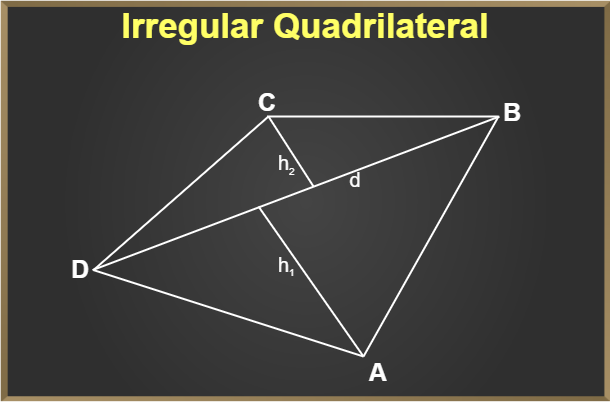From the figure, the area of the quadrilateral ABCD = area of ΔABD + area of ΔBCD.

Area of the quadrilateral ABCD = (1/2) × d × h1+ (1/2) × d × h2 = (1/2) × d ×( h1+h2 ).

Thus, the formula used to find the area of a quadrilateral is,

Area of Quadrilateral = (1/2) × Diagonal × (Sum of heights) = (1/2) × d ×( h1+h2 )

## Area of Quadrilateral with Vertices

If vertices of a quadrilateral are given then its area is calculated by the given formula. Suppose  A(x1, y1), B(x2, y2), C(x3, y3), and D(x4, y4) be the vertices of a quadrilateral ABCD.

Then its area is calculated by using two different methods which are discussed below: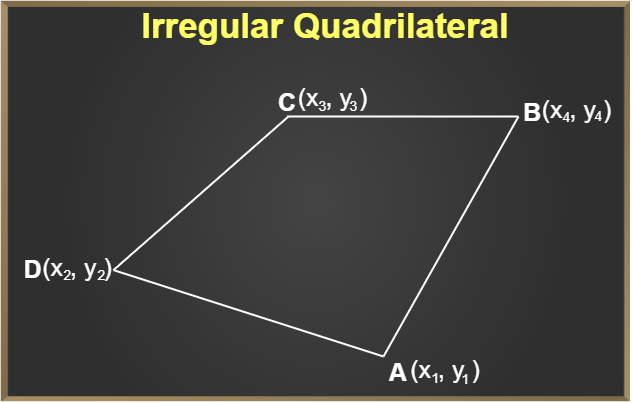## Area of Quadrilateral Using Coordinates (Method 1)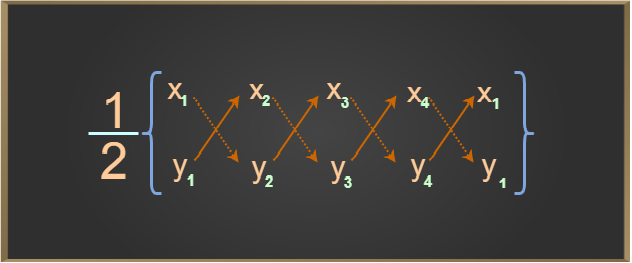Follow the directions of the arrow, and add the diagonal products, i.e., x1y2, x2y3, x3y4, and x4y1.

(x1y2 + x2y3 + x3y4 + x4y1)….(i)

Now, follow the dotted arrows and add the diagonal products, i.e., x2y1, x3y2, x4y3, and x1y4.

(x2y1 + x3y2 + x4y3 + x1y4)….(ii)

Now, subtract equation (ii) from (i) and multiply the result by 1/2.

(1/2) × [(x1y2 + x2y3 + x3y4 + x4y1) – (x2y1 + x3y2 + x4y3 + x1y4)]

Thus, the formula for the area of quadrilateral when vertices are given:

Area of Quadrilateral = (1/2) × [(x1y2 + x2y3 + x3y4 + x4y1) – (x2y1 + x3y2 + x4y3 + x1y4)]

## Area of Quadrilateral Using Heron’s Formula (Method 2)

For this method, we divide the given quadrilateral into two triangles and then find the area of each triangle separately using Heron’s formula. At last, both the area of triangles are added to find the final area of the quadrilateral.

Area of quadrilateral ABCD = Area of triangle ABD + Area of triangle BCD

Area of a triangle with vertices P(x1, y1), Q(x2, y2), and R(x3, y3) is given by

Area of Δ = (1/2) × {x1(y2 – y3) + x2(y3 – y1) + x3(y1 – y2)}

## How to find the Area of a Quadrilateral?

Area of a quadrilateral is found by using the steps discussed below:

Step 1: Mark the length of the diagonal and the length of the perpendicular to it from both the vertices.

Step 2: Put these values in the given formula Area = (1/2) × d ×( h1+h2 ), where d is the length of the diagonal and h1, h2 are lengths of the perpendicular from diagonal to opposite vertices.

Step 3: Answer obtained from the above step is the required area and is measured in unit2

## Area of Some Quadrilaterals

Some specific quadrilaterals are very common and are used in our daily life and their formula for areas are explained in the article given below:

### Area of a Rectangle

A rectangle is a closed figure having four sides in which opposite sides are equal and parallel to each other and the diagonals of the rectangles bisect at 90 degrees.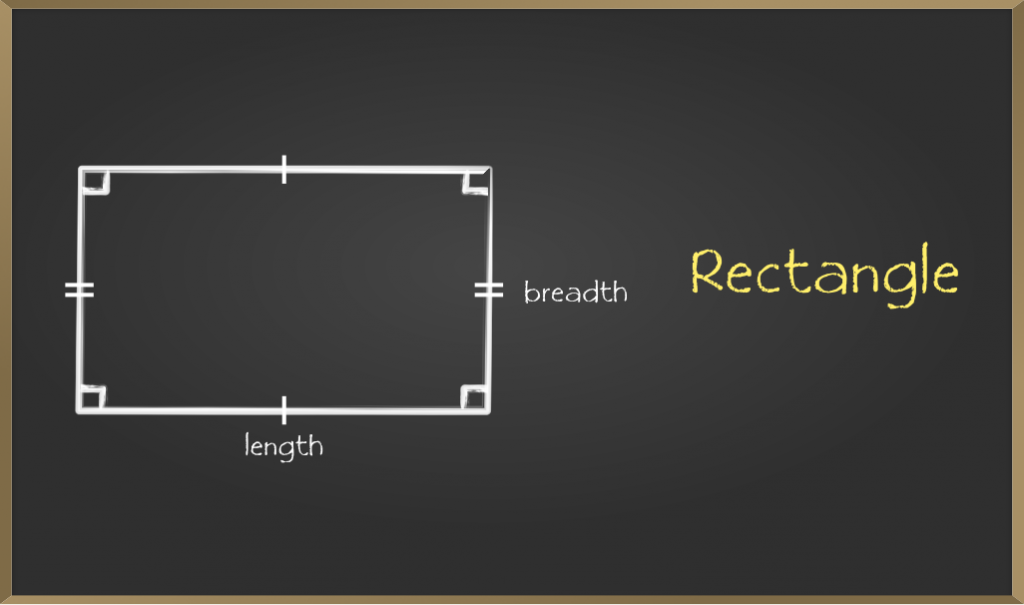Area of Rectangle = (Length x Breadth) m2

### Area of a Square

A square is a special case of a rectangle in which the four sides are equal and all the sides are parallel to each other. In a square diagonal bisect perpendicularly to each other.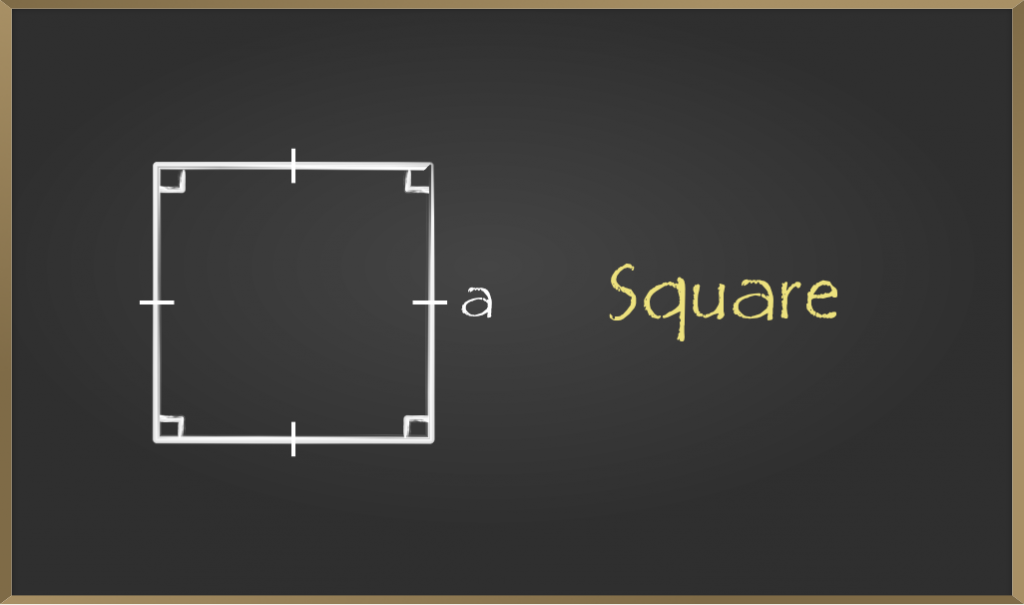Area of  Square =  (side x side ) m2

### Area of Rhombus

A Rhombus is a special case of the square in which all the four sides and opposite angles are the same in measure and the opposite sides are parallel and the sum of the adjacent angles of a rhombus is equal to 180 degrees.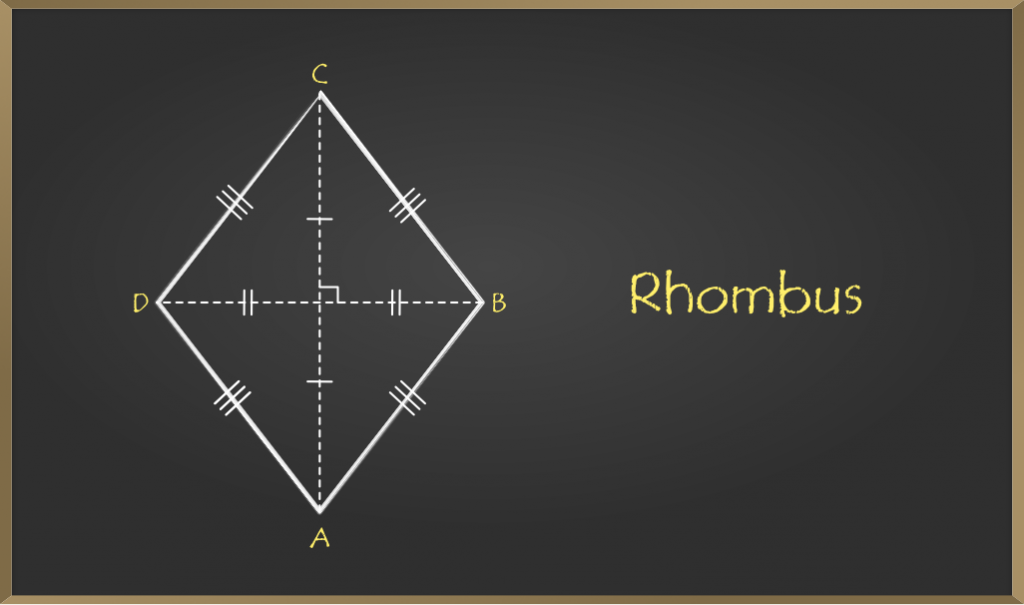Area of Rhombus = (1/2) x D1 x D2 m2

### Area of Parallelogram

The quadrilateral in which opposite sides are equal and parallel to each other is known as a parallelogram. In this, diagonals bisect each other and the opposite angles are of equal measure in which the sum of two adjacent angles of a parallelogram is equal to 180 degrees.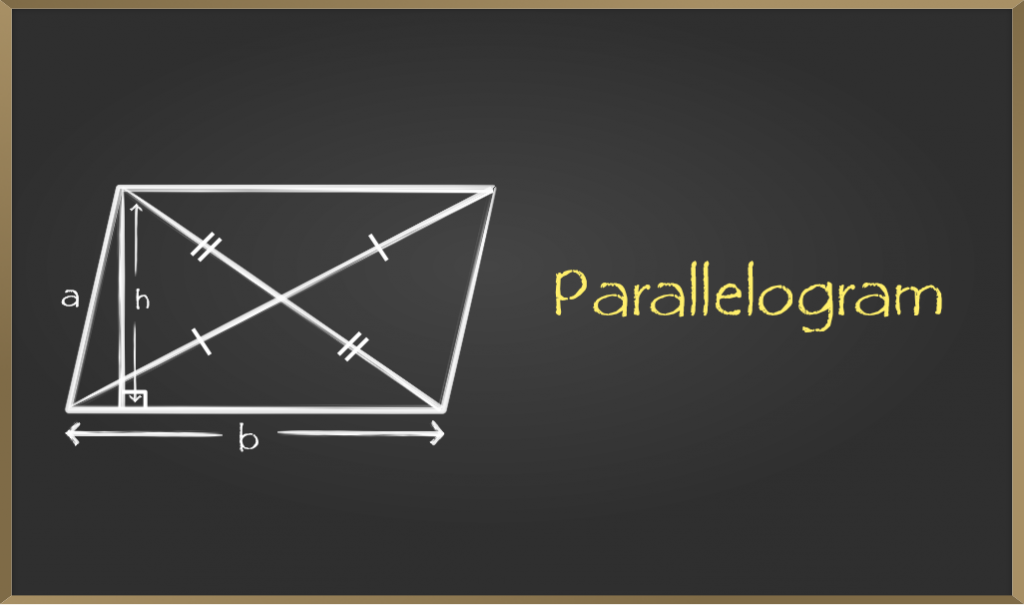Area of Parallelogram = (Base x Height) m2

### Area of Trapezium

This quadrilateral is somewhat different from the others as there is only one pair of the opposite side of a trapezium parallel to each other and the adjacent sides are supplementary to each other and the diagonals bisect each other in the same ratio.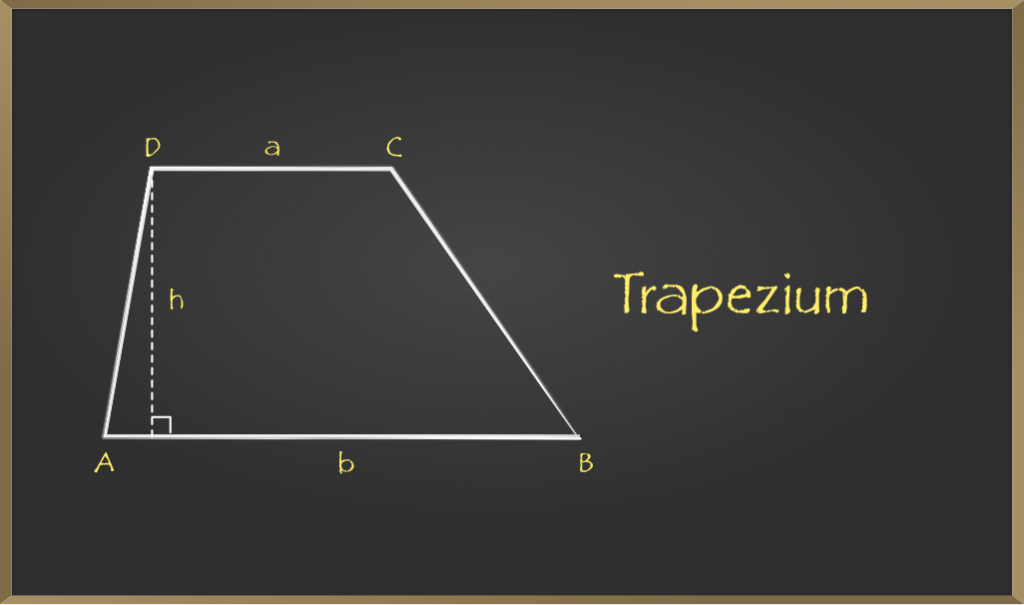Area of Trapezium = (1/2) h (AB+CD) m2

### Area of Kite

Kite is a special quadrilateral in which each pair of consecutive sides is congruent, but the opposite sides are not congruent. In this, the largest diagonal of a kite bisects the smallest diagonal.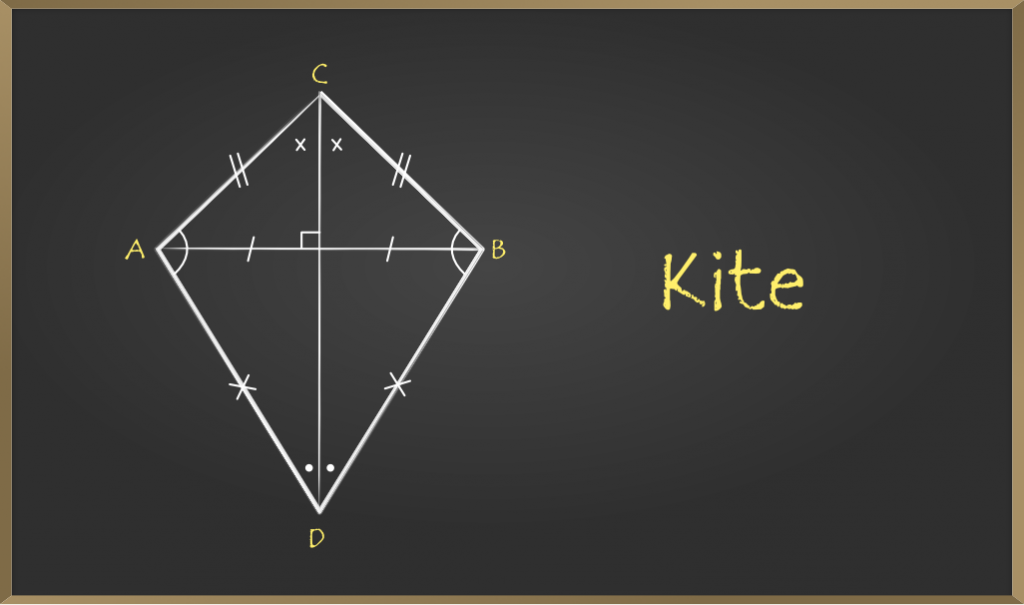Area of kite =1/2 x D1 x D2  m2

where,
D1 = long diagonal of kite(KM)
D2 = short diagonal of kite(JL)

## Solved Example on Area of Quadrilateral

Example 1: Find the area of the quadrilateral ABCD when its vertices are (1, 2), (5, 6), (4, −6), and (−5, 2).

Solution:

Let A(1, 2), B(5, 6), C(4, -6), and D(-5, 2) be the vertices of a quadrilateral ABCD.

A(1, 2) = (x1, y1), B(5, 6) = (x2, y2), C(4, -6) = (x3, y3), D(-5, 2) = (x4, y4)

We know that,

Area of Quadrilateral = (1/2) × [(x1y2 + x2y3 + x3y4 + x4y1) – (x2y1 + x3y2 + x4y3 + x1y4)]

= (½). {[1(6) + 5(-6) + 4(2) + (-5)2] – {[5(2) + 4(6) + (-5)(-6) + 1(2)]}

= (½).[(6 – 30 + 8 – 10) – (10 + 24 + 30 + 2)]

= (½) [-26 – 66]

= 92/2 (area is never negative)

= 46 unit2

Example 2: Find the area of trapezium if height is 5 cm and AB and CD are given as 10 and 6 cm respectively.

Solution:

Given, AB = 10cm, CD = 6cm, height = 5cm

According to the formulae,

Area of Trapezium = (1/2) h (AB+CD)

= 1/2 x 5 x (10 + 6)

Area of Trapezium = 40 cm2

Example 3: Find the area of a kite whose longest and shortest diagonals are 20cm and 10cm respectively.

Solution:

Length of longest diagonal, D1= 20 cm

Length of shortest diagonal, D2= 10 cm

So,

Area of kite =1/2 x D1 x D2

Area of kite = 1/2 x 20 x 10 = 100 cm2

Example 4: Calculate the area of a parallelogram, if the base and height are 10 m and 15 m respectively.

Solution:

Given, base = 10 m and height = 15 m

Area of Parallelogram = Base x Height

Area of Parallelogram = 10 x 15

Area of Parallelogram = 150 m2

Example 5: Given the area of the rhombus is 120-meter square then find the length of one of the diagonals if the other diagonal is of length 12 m.

Solution:

Since we know that,

Area of Rhombus = (1/2) x Diagonal 1 x Diagonal 2

Putting all the known values, we get

120 = (1/2) x Diagonal 1 x Diagonal 2

Diagonal 2 = 20 m

## FAQs on Area of Quadrilateral

Question 1: What is the area of a quadrilateral?

Area of the quadrilateral is the region inside the boundry of a quadrilateral. It is the total space occupied by a quadrilateral in 2-D plane. It is measured in square units.

Question 2: How to find the area of a quadrilateral?

Area of quadrilateral is found using formula given below:

Area of Quadrilateral = (1/2) × [(x1y2 + x2y3 + x3y4 + x4y1) – (x2y1 + x3y2 + x4y3 + x1y4)]

where (x1, y1), (x2, y2), (x3, y3), and (x4, y4) are the vertices of a quadrilateral.

Question 3: What are the different types of quadrilaterals?

Different types of quadrilateral are:

• Square
• Rectangle
• Rhombus
• Kite
• Parallelogram
• Trapezium

Question 4: Write the uses of quadrilaterals.

Area of quadrilateral is used in the field of architecture, agriculture, design, and navigation also it helps to find distance between two points. It is required to find the area of bulidings, park and other complexes.

Question 5: How to calculate the area of a quadrilateral if one of its diagonals and both perpendiculars from the vertices are given?

When the diagonal(d) and the length of both perpendiculars (h, H) from the vertices are given, then the area of the quadrilateral is calculated by the formula:

Area of quadrilateral = (½) × d × (h + H)

Question 6: What are the two main types of quadrilaterals?

The two main types of a quadrilateral are

Question 7: How to find the Area of a Quadrilateral using Heron’s Formula?

To find area of triangle using Heron’s Formula use the following steps:

Step 1: Divide the quadrilateral in two triangles by joining its diagonal.

Step 2: Find the area of both triangles using Heron’s formula.

Step 3: Add both the areas to get the final answer.

### Related Resources

My Personal Notes arrow_drop_up